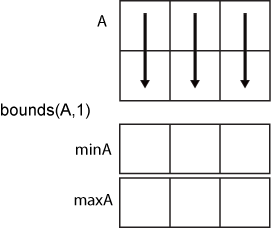# bounds

Smallest and largest elements

## Syntax

``````[S,L] = bounds(A)``````
``[S,L] = bounds(A,'all')``
``````[S,L] = bounds(A,dim)``````
``[S,L] = bounds(A,vecdim)``
``````[S,L] = bounds(___,nanflag)``````

## Description

example

``````[S,L] = bounds(A)``` returns the smallest element `S` and largest element `L` of an array. `S` is equivalent to `min(A)` and `L` is equivalent to `max(A)`.```

example

````[S,L] = bounds(A,'all')` computes the smallest and largest values over all elements of `A`. This syntax is valid for MATLAB® versions R2018b and later.```

example

``````[S,L] = bounds(A,dim)``` operates along the dimension `dim` of `A`. For example, if `A` is a matrix, then `bounds(A,2)` returns column vectors `S` and `L` containing the smallest and largest elements of each row.```

example

````[S,L] = bounds(A,vecdim)` computes the smallest and largest values based on the dimensions specified in the vector `vecdim`. For example, if `A` is a matrix, then `bounds(A,[1 2])` returns the smallest and largest values over all elements in `A`, since every element of a matrix is contained in the array slice defined by dimensions 1 and 2.```

example

``````[S,L] = bounds(___,nanflag)``` specifies whether to include or omit `NaN` values when determining the smallest and largest elements. `bounds(A,'omitnan')` ignores `NaN` values. If any element of `A` is `NaN`, then `bounds(A,'includenan')` returns `NaN` for both `S` and `L`. The default behavior is `'omitnan'`.```

## Examples

collapse all

Simultaneously compute the smallest and largest values of a vector.

```A = [2 4 -1 10 6 3 0 -16]; [S,L] = bounds(A)```
```S = -16 ```
```L = 10 ```

Compute the smallest and largest elements of each row of a matrix.

`A = magic(4)`
```A = 4×4 16 2 3 13 5 11 10 8 9 7 6 12 4 14 15 1 ```
`[S,L] = bounds(A,2)`
```S = 4×1 2 5 6 1 ```
```L = 4×1 16 11 12 15 ```

Create a 3-D array and compute the smallest and largest values in each page of data (rows and columns).

```A(:,:,1) = [2 4; -2 1]; A(:,:,2) = [9 13; -5 7]; A(:,:,3) = [4 4; 8 -3]; [S1,L1] = bounds(A,[1 2]); S1```
```S1 = S1(:,:,1) = -2 S1(:,:,2) = -5 S1(:,:,3) = -3 ```
`L1`
```L1 = L1(:,:,1) = 4 L1(:,:,2) = 13 L1(:,:,3) = 8 ```

Starting in R2018b, to compute the bounds over all dimensions of an array, you can either specify each dimension in the vector dimension argument, or use the `'all'` option.

`[S2,L2] = bounds(A,[1 2 3])`
```S2 = -5 ```
```L2 = 13 ```
`[Sall,Lall] = bounds(A,'all')`
```Sall = -5 ```
```Lall = 13 ```

Include and ignore NaN elements of a vector when computing its smallest and largest values.

Ignore `NaN` values when computing the largest and smallest values of a vector, which is the default.

```A = [2 NaN 6 -5 0 NaN 10]; [S,L] = bounds(A)```
```S = -5 ```
```L = 10 ```

Use the `'includenan'` option to include `NaN` values, which causes `bounds` to return `NaN` for both the smallest and largest values of `A`.

`[S,L] = bounds(A,'includenan')`
```S = NaN ```
```L = NaN ```

## Input Arguments

collapse all

Input array, specified as a vector, matrix, or multidimensional array.

Data Types: `single` | `double` | `int8` | `int16` | `int32` | `int64` | `uint8` | `uint16` | `uint32` | `uint64` | `logical` | `categorical` | `datetime` | `duration`
Complex Number Support: Yes

Dimension to operate along, specified as a positive integer scalar. If no value is specified, then the default is the first array dimension whose size does not equal 1.

Consider a matrix `A`.

• `bounds(A,1)` computes the smallest and largest values of each column.• `bounds(A,2)` computes the smallest and largest values of each row.Data Types: `double` | `single` | `int8` | `int16` | `int32` | `int64` | `uint8` | `uint16` | `uint32` | `uint64`

Vector of dimensions, specified as a vector of positive integers. Each element represents a dimension of the input array. The lengths of the output in the specified operating dimensions are 1, while the others remain the same.

Consider a 2-by-3-by-3 input array, `A`. Then `[S,L] = bounds(A,[1 2])` returns a 1-by-1-by-3 array for both `S` and `L`. The elements of `S` and `L` are the smallest and largest values in the corresponding page of `A`, respectively.Data Types: `double` | `single` | `int8` | `int16` | `int32` | `int64` | `uint8` | `uint16` | `uint32` | `uint64`

`NaN` condition, specified as one of these values:

• `'omitnan'` — Ignore all `NaN` values in the input. If the input contains only `NaN` values, then `bounds` returns `NaN` for both `S` and `L`.

• `'includenan'` — Include `NaN` values. If any element of the input is `NaN`, then `bounds` returns `NaN` for both `S` and `L`.

## Output Arguments

collapse all

Smallest element, specified as a vector, matrix, or multidimensional array.

Largest element, specified as a vector, matrix, or multidimensional array.